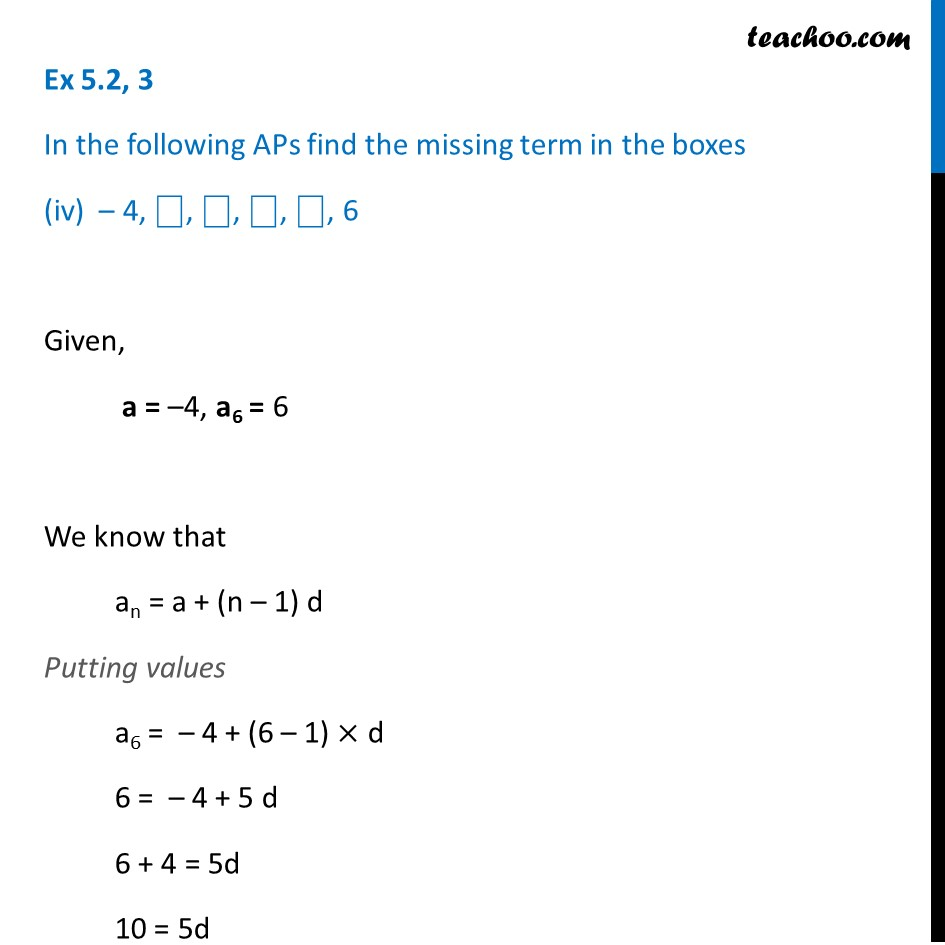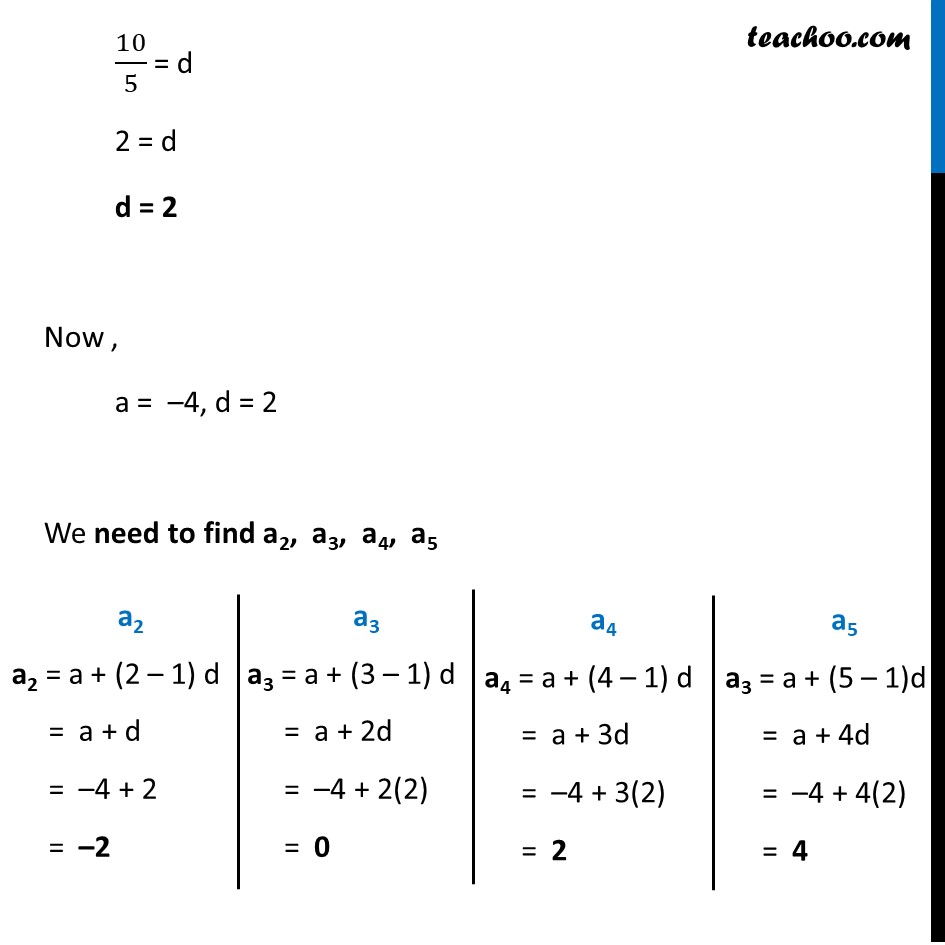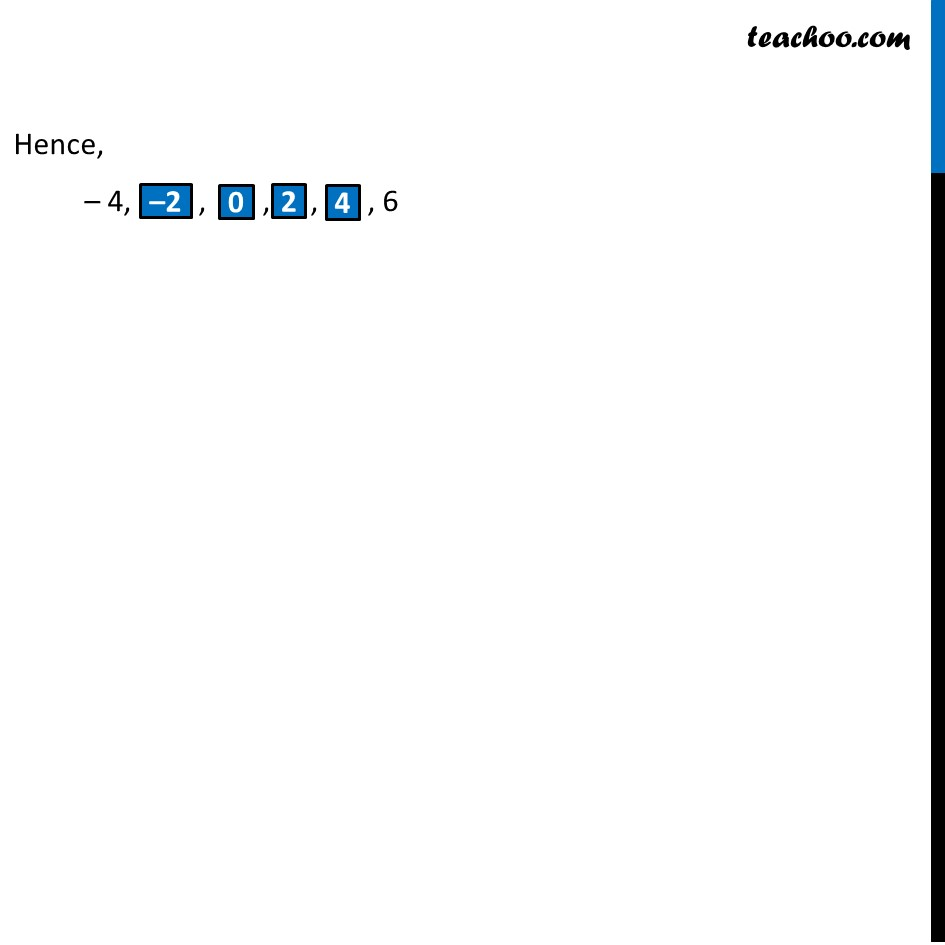1. Chapter 5 Class 10 Arithmetic Progressions (Term 2)
2. Serial order wise
3. Ex 5.2

Transcript

Ex 5.2, 3 In the following APs find the missing term in the boxes (iv) – 4, ⎕, ⎕, ⎕, ⎕, 6 Given, a = –4, a6 = 6 We know that an = a + (n – 1) d Putting values a6 = – 4 + (6 – 1) × d 6 = – 4 + 5 d 6 + 4 = 5d 10 = 5d 10/5 = d 2 = d d = 2 Now , a = –4, d = 2 We need to find a2, a3, a4, a5 a2 a2 = a + (2 – 1) d = a + d = –4 + 2 = –2 a3 a3 = a + (3 – 1) d = a + 2d = –4 + 2(2) = 0 a4 a4 = a + (4 – 1) d = a + 3d = –4 + 3(2) = 2 a5 a3 = a + (5 – 1)d = a + 4d = –4 + 4(2) = 4 Hence, – 4, , , , , 6